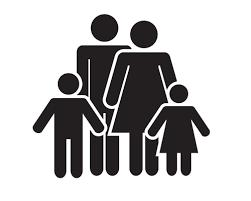# Family 8

Father is 38 years old, daughter 12, son 14. How many years will father have as many years as his children together?

x =  12

### Step-by-step explanation:

38+x = (12+x) + (14+x)

x = 12

Our simple equation calculator calculates it.Did you find an error or inaccuracy? Feel free to write us. Thank you!

Showing 1 comment:
Math student
I still have no idea how to you even got this because i haven't learned this yet and i don't even know why im searching it up please don't ask why

Tips for related online calculators
Do you have a linear equation or system of equations and looking for its solution? Or do you have a quadratic equation?
Do you want to convert time units like minutes to seconds?